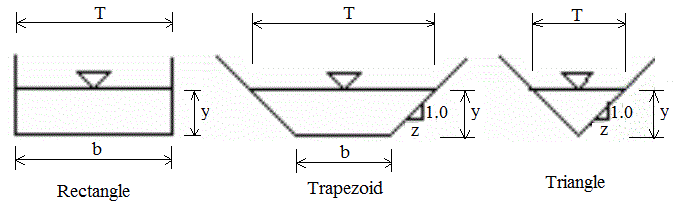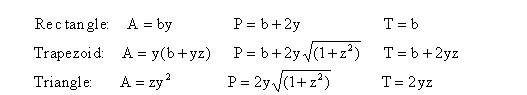Open Channel

Open channel hydraulic radius, wetted perimeter, area

 Select open channel: Rectangular Cross-Section Trapezoidal Cross-Section Triangular Cross-Section Depth, y (L): © 2014 LMNO Engineering, Bottom Width, b (L): Research, and Software, Ltd. Side Slope, z (H:V): http://www.LMNOeng.com Area, A (L2): Wetted Perimeter, P (L): Hydraulic Radius, R (L): Top Width, T (L):

Open channel units: Any units in the open channel calculator may be used, so long as they are consistent. (L) means that the variable has units of length (e.g. meters). (L2) means that the variable has units of length squared (e.g. m2) in the open channel calculator.

Open Channel Diagram and Equations:In open channel, z is side slope of trapezoidal and triangular channels, defined as horizontal to vertical ratio. Both sides of the open channel are assumed to have the same side slope.

Open Channel Equations (note that R=A/P):The non-circular open channel geometry calculation does not check for unreasonable inputs. Please enter positive values.

LMNO Engineering, Research, and Software, Ltd.
7860 Angel Ridge Rd.   Athens, Ohio 45701  USA   Phone: (740) 592-1890
LMNO@LMNOeng.com    http://www.LMNOeng.com

To:

Manning Equation Calculator

Design of Trapezoidal Open Channels Calculator

Design of Rectangular Open Channels Calculation

Unit Conversions

Other information:

Register

Discussion and References